# DAV Class 3 Maths Chapter 2 Worksheet 2 Solutions

The DAV Class 3 Maths Book Solutions and DAV Class 3 Maths Chapter 2 Worksheet 2 Solutions of Addition offer comprehensive answers to textbook questions.

## DAV Class 3 Maths Ch 2 WS 2 Solutions

Question 1.

(a)Solution:(b)Solution: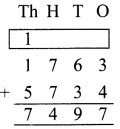(c)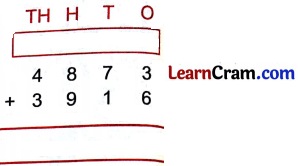Solution: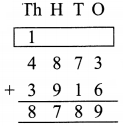(d)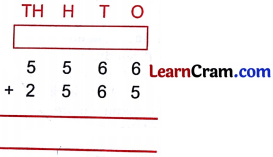Solution:(e)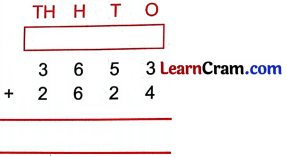Solution:(f)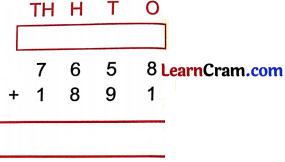Solution: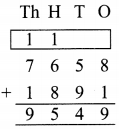(g)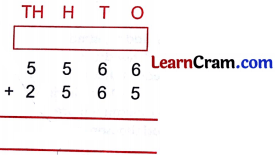Solution: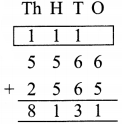(h)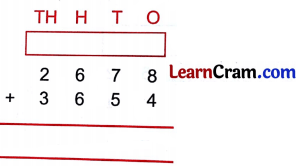Solution:(i)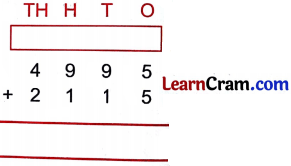Solution:(j)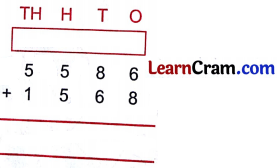Solution: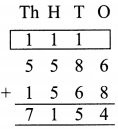(k)Solution: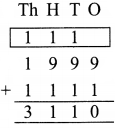(l)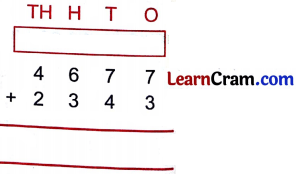Solution:Question 2.
Arrange in columns and add the following:

(a) 3875, 4824
Solution: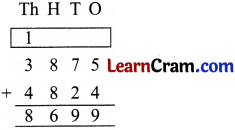(b) 7655, 1155
Solution:(c) 5557, 2565
Solution: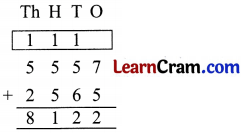(d) 5746, 1228
Solution: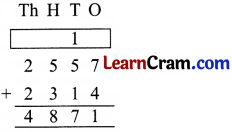(e) 2557, 2314
Solution: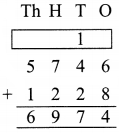(f) 6769, 3211
Solution: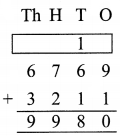### DAV Class 3 Maths Chapter 2 Worksheet 2 Notes

Remember :

3. Carry 1 to thousand place and add

Example:Similarly: